# 整理的90个公式,学完你就能碾压99%的同事

全套总共90个案例，超级全面。今天先分享15个过100个。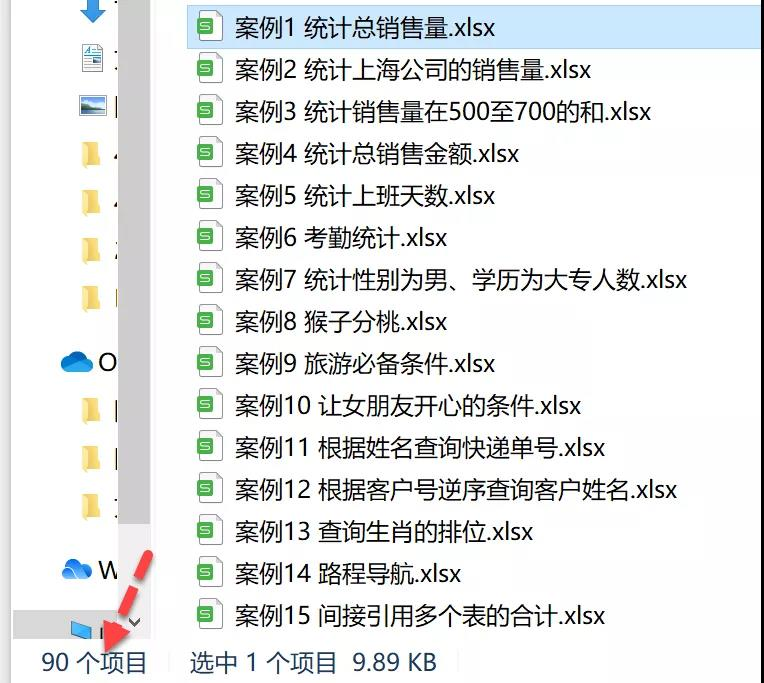1.统计总销售量（SUM函数）

=SUM(C2:C11)SUM(数字1,[数字2],……)

2.

=SUMIF(B:B,E2,C:C)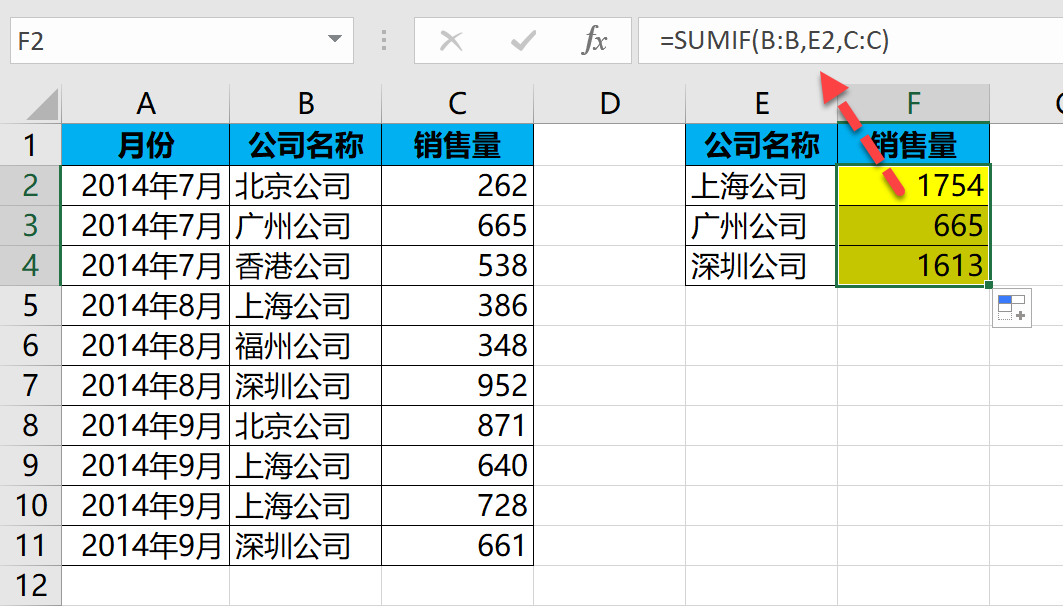SUMIF函数语法如下：

SUMIF(条件区域,条件,[求和区域])

=SUMIF(C:C,">500",C:C)

=SUMIF(C:C,">500")

3.

500至700的和（SUMIFS函数）

=SUMIFS(C:C,C:C,">=500",C:C,"<=700")SUMIFS(求和区域,条件区域1,条件1,[条件区域2],[条件2],……)

SUMIFS跟SUMIF的参数顺序有点不同，SUMIFS求和区域在第一参数，而SUMIF函数求和区域在第三参数，这个需要特别注意一 下。

4.

SUMPRODUCT函数）

=SUMPRODUCT(D2:D11,E2:E11)SUMPRODUCT(区域1, 区域2, ……)

5.

=COUNTA(B3:C33)/2COUNTA函数语法如下：

COUNTA(值1,[值2],……)

COUNTA函数语法跟SUM函数一样。

6.

COUNTIF函数）

=COUNTIF(\$B2:\$I2,J\$1)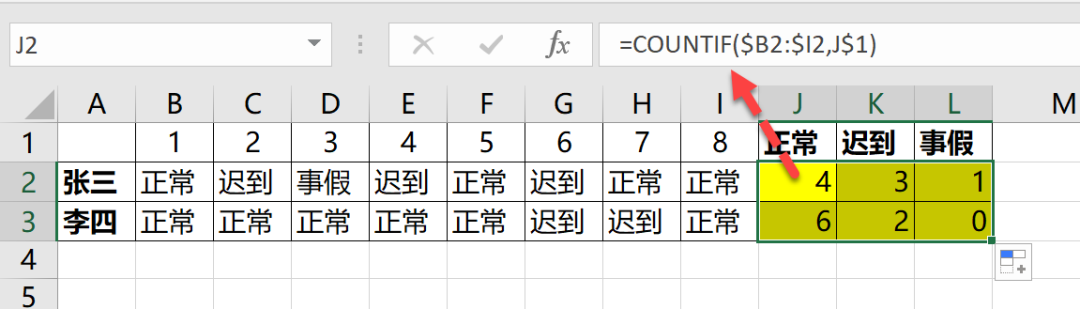COUNTIF函数就是条件计数函数，跟SUMIF函数有点类似，就是少了一个求和区域而已。

COUNTIF函数语法：

COUNTIF(条件区域,条件)

7.

=COUNTIFS(C2:C11,"男",E2:E11,"大专")COUNTIFS函数可以实现多条件计数，函数语法如下：

COUNTIFS(条件区域1,条件1, 条件区域2,条件2,……)

8.

1．如果是早上就给3个桃子，否则（是晚上）就给4个

2．如果是早上就给4个桃子，否则（是晚上）就给3个

=IF(B2="早上",3,4)=IF(B2="早上",4,3)IF函数的作用就是进行逻辑判断，语法如下：

IF(条件,条件符合时返回的值,条件不符合时返回的值)

9.

AND函数）

=IF(AND(A2="有钱",B2="有时间"),"出去玩","不玩")AND函数的作用就是同时满足的意思，语法如下：

AND(条件1,条件2,……)

10.

=IF(OR(A2="吃大餐",B2="送礼物",C2="安慰她",D2="讲趣事",E2="去散心",F2="陪伴"),"开心","不开心")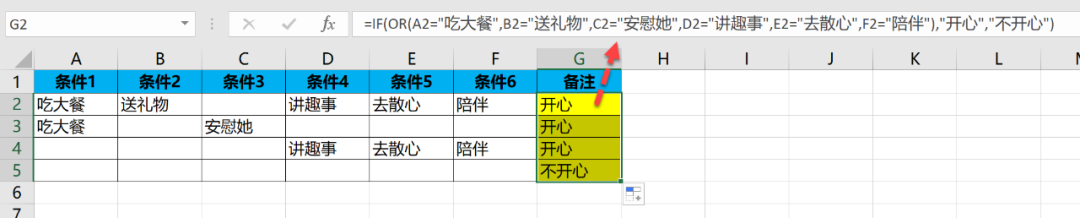OR函数就是或者的意思，语法如下：

OR(条件1,条件2,……)

11.

=VLOOKUP(D2,A:B,2,0)&""VLOOKUP(查找值,查找区域,返回查找区域的第几列,精确还是模糊查找)

12.

LOOKUP函数）

=LOOKUP(1,0/(\$B\$2:\$B\$34=D2),\$A\$2:\$A\$34)LOOKUP函数经典查找模式通用公式：

LOOKUP(1,0/((条件1)*(条件2)*……*(条件n)),返回区域)

13.

MATCH函数）

=MATCH(C2,A2:A13,0)MATCH函数的语法如下：

MATCH(查找值,查找的区域,精确或者模糊查找)

14.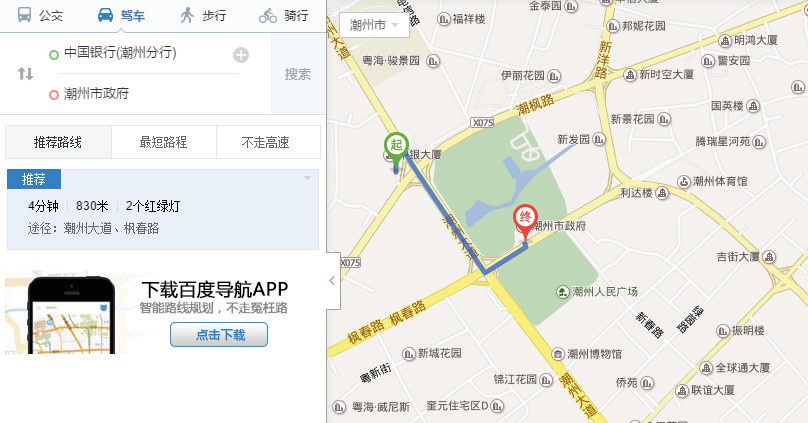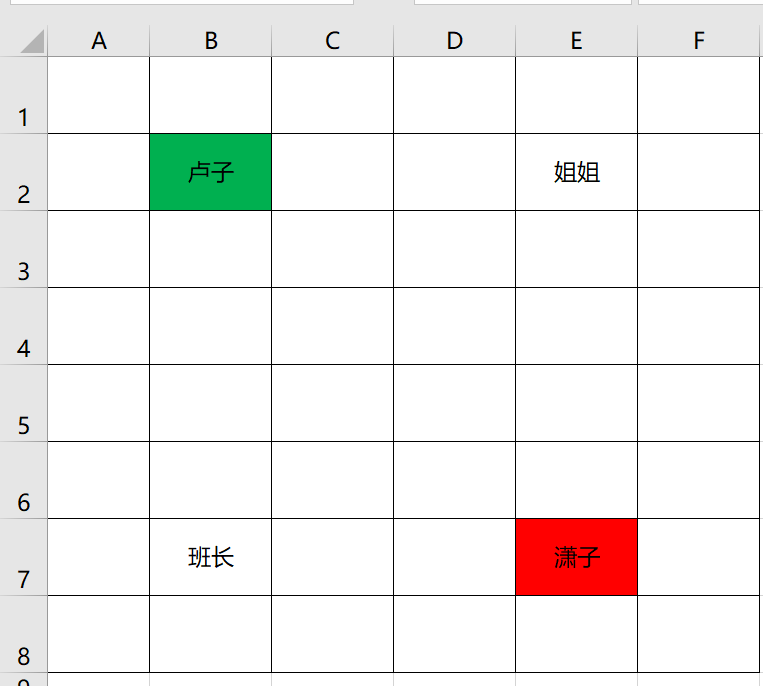1．往右边走3站到姐姐家，再往下边走5站到潇子家。

2．往下边走5站到班长家，再往右边走3站到潇子家。只往右边走3站，不向下走。

=OFFSET(B2,5,3)

OFFSET函数的语法如下：

OFFSET(起点,向下多少行,向右多少列)

=OFFSET(E7,-5,-3)

15.

INDIRECT函数）

1月到4月机台的产量，4个表格格式一模一样，如何引用所有表格的合计呢？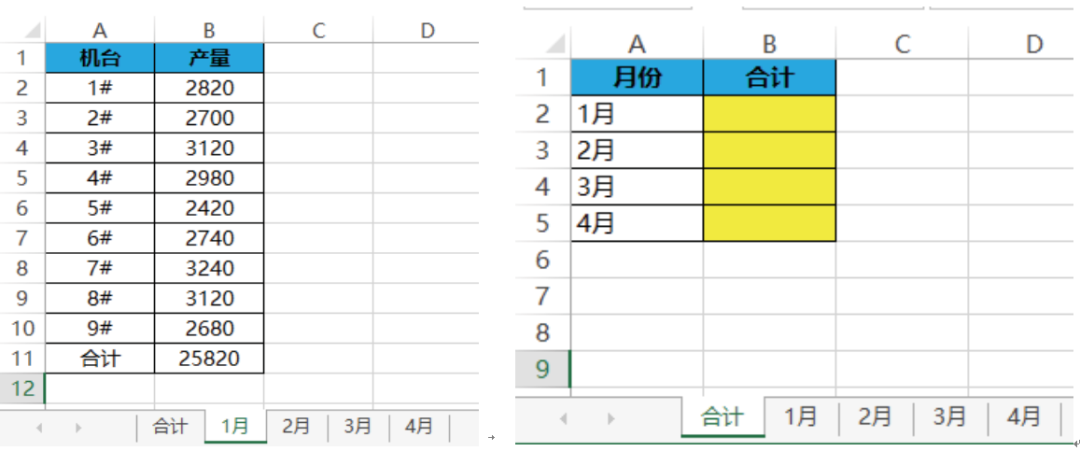=INDIRECT(A2&"!B11")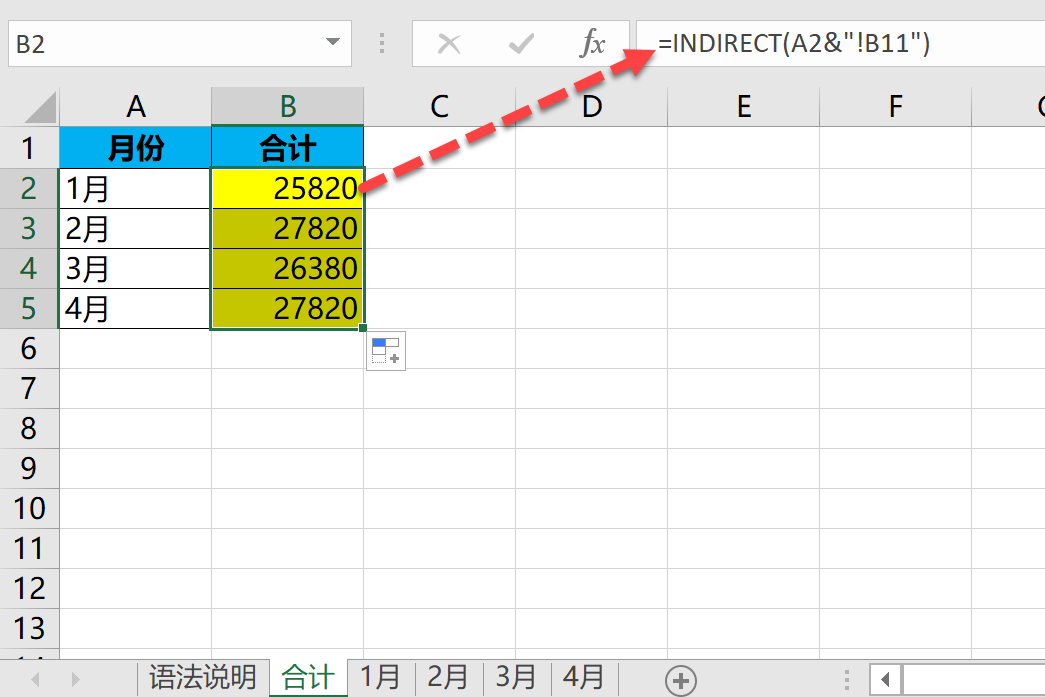INDIRECT函数的语法如下：

INDIRECT(单元格引用)